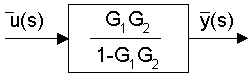Summary Week 6
1. Method of Partial Fractions to find inverse Laplace transform

2.

Given:  f(s) = num(s)/den(s)

where num(s)=numerator polynomial in s, den(s)=denominator polynomial in s

Step 1: Find the roots of the denominator polynomial, say  - r1,  ... ,  -rN

Step 2: Based on these roots separate num(s)/den(s) into partial fractions.

Case 1: For each root, say -q, appearing k times, add the following k terms: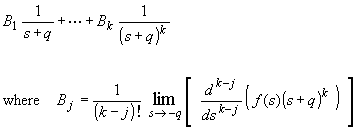Case 2: For each complex pair of roots, say -a+ib and -a-ib, add two terms: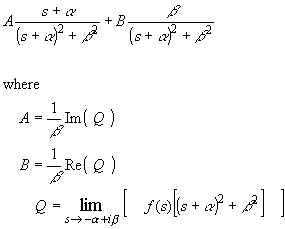Step 3. If needed, take the inverse Laplace transforms of each terms using the following formulas:3. Transfer Functions

4.

Definition: a relationship from the input variable, say u, to an output variable, say y, in the Laplace domain

Function Form: L(y) = G(s) L(u), where G(s) is the transfer function

Block Diagram Form: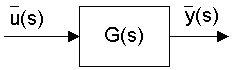5. Equivalent Transfer Functions
a ) Series: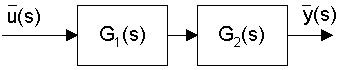equivalent to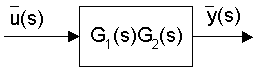b ) Parallel Sum: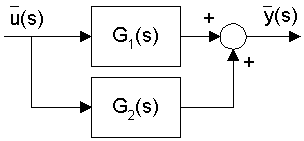equivalent to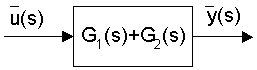c) Feedback (Positive):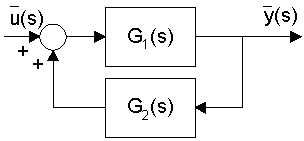equivalent to